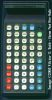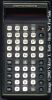Datasheet legend
Ab/c: Fractions calculation
AC: Alternating current
BaseN: Number base calculations
Card: Magnetic card storage
Cmem: Continuous memory
Cond: Conditional execution
Const: Scientific constants
Cplx: Complex number arithmetic
DC: Direct current
Eqlib: Equation library
Exp: Exponential/logarithmic functions
Fin: Financial functions
Grph: Graphing capability
Hyp: Hyperbolic functions
Intg: Numerical integration
Jump: Unconditional jump (GOTO)
Lbl: Program labels
LCD: Liquid Crystal Display
LED: Light-Emitting Diode
Li-ion: Lithium-ion rechargeable battery
Lreg: Linear regression (2-variable statistics)
mA: Milliamperes of current
Mtrx: Matrix support
NiMH: Nickel-metal-hydrite rechargeable battery
Prnt: Printer
RTC: Real-time clock
Sdev: Standard deviation (1-variable statistics)
Solv: Equation solver
Subr: Subroutine call capability
Symb: Symbolic computing
Tape: Magnetic tape storage
Trig: Trigonometric functions
Units: Unit conversions
VAC: Volts AC
VDC: Volts DC
 Years of production: Display type: 7-segment New price: Display color: Red Display technology: LED Size: 6"×3"×1½" Display size: 10+2 digits Weight: 8 oz Entry method: Algebraic Batteries: 3×"AA" sealed NiCd Advanced functions: trg, hyp, exp, log, drg, dms, stat, prob, complex External power: Commodore adapter Memory functions: + I/O: N/A Programming model: N/A Precision: 12 digits Program functions: N/A Memories: SR-4190: 2, SR-9190: 10 Program display: N/A Program memory: N/A Program editing: N/A Chipset: Forensic result:While not a programmable beast, this calculator is one of the most versatile early scientific calculators I've ever seen. In fact, it was this calculator (the very machine pictured here, actually) that got me started on my quest to write an efficient and accurate Gamma function implementation on the programmables I later owned.

In addition to the usual complement of scientific and statistical functions and a pair of storage registers, this calculator has complex arithmetic, three probability distribution functions (Gaussian, Poisson, and binomial), a large number of unit conversions, and of course the infamous Gamma function that I was so obsessed with.

The calculator displays results with ten digits of precision, but uses 12 digits internally. This leads to an interesting quirk. If you have one of these calculators on hand, try typing the following:

9.999999999 EE 99 + 9.9 EE 89 =

The result is 1. But this is a strange kind of 1; take its square root, for instance, and you get 1e50. What happens is that the calculator rounds up 9.99999999999e99 to 1e100 before display. However, since the exponent can only contain two digits, the result in fact becomes 1e00, or 1. Other calculators display an error when this condition occurs, but not the SR-4190R. Peculiar, isn't it?

I received this calculator from a good friend in Hungary many years ago (the same friend who owned it back in 1977 or so, when I first saw it.) With a new set of NiCad batteries, the calculator still works perfectly. In fact, I often use it to verify the accuracy of my various Gamma function programs on other vintage calculators!I have since acquired another Commodore machine, a close relative of the SR-4190R: the SR-9190R. Apart from a different color scheme, the two calculators look very similar in appearance. However, the SR-9190R has not only many more memories (10 in place of the SR-4190R's two), it also sports a unique feature: instead of the Gamma function, it calculates its natural logarithm, which of course yields values that the calculator can display for a far broader range of arguments, making it that much more practical for use with real-life problems.